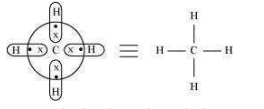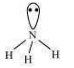9.8 What do you understand by (i) electron-deficient, (ii) electron-precise, and (iii) electron-rich compounds of hydrogen? Provide justification with suitable examples.

Molecular hydrides are classified on the basis of the presence of the total number of electrons and bonds in their Lewis structures as:

1. Electron-deficient hydrides

2. Electron-precise hydrides

3. Electron-rich hydrides
An electron-deficient hydride has very few electrons, less than that required for representing its conventional Lewis structure e.g. diborane (${B}_{2}{H}_{6}$). In ${B}_{2}{H}_{6}$, there are six bonds in all, out of which only four bonds are regular two centered-two electron bonds. The remaining two bonds are three centered-two electron bonds i.e., two electrons are shared by three atoms. Hence, its conventional Lewis structure cannot be drawn. An electron-precise hydride has a sufficient number of electrons to be represented by its conventional Lewis structure e.g. $C{H}_{4}$. The Lewis structure can be written as:Four regular bonds are formed where two electrons are shared by two atoms.
An electron-rich hydride contains excess electrons as lone pairs e.g. $N{H}_{3}$.There are three regular bonds in all with a lone pair of electrons on the nitrogen atom.